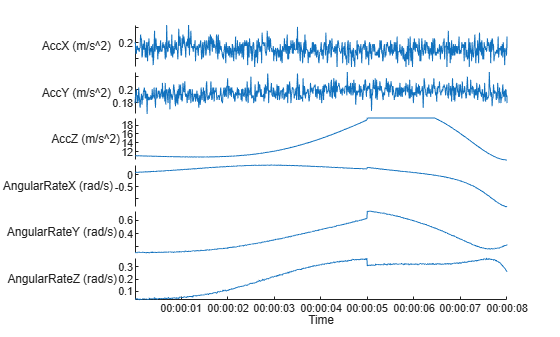createCustomSensorTemplate

Create sample implementation for UAV custom sensor interface

Description

example

createCustomSensorTemplate creates a sample implementation for UAV custom sensor that inherits from the uav.SensorAdaptor class. This function opens a new file in the MATLAB® Editor.

Examples

collapse all

Create a sensor adaptor for an imuSensor from Navigation Toolbox™ and gather readings for a simulated UAV flight scenario.

Use the createSensorAdaptorTemplate function to generate a template sensor and update it to adapt an imuSensor object for usage in UAV scenario.

createCustomSensorTemplate

This example provivdes the adaptor class uavIMU, which can be viewed using the following command.

edit uavIMU.m

Use Sensor Adaptor in UAV Scenario Simulation

Use the IMU sensor adaptor in a UAV Scenario simulation. First, create the scenario.

scenario = uavScenario("StopTime", 8, "UpdateRate", 100);

Create a UAV platform and specify the trajectory. Add a fixed-wing mesh for visualization.

plat = uavPlatform("UAV", scenario, "Trajectory", ...
waypointTrajectory([0 0 0; 100 0 0; 100 100 0], "TimeOfArrival", [0 5 8], "AutoBank", true));
updateMesh(plat,"fixedwing", {10}, [1 0 0], eul2tform([0 0 pi]));

Attach the IMU sensor using the uavSensor object and specify the uavIMU as an input. Load parameters for the sensor model.

imu = uavSensor("IMU", plat, uavIMU(imuSensor));

fn = fullfile(matlabroot,'toolbox','shared',...
'positioning','positioningdata','generic.json');

Visualize the scenario.

figure
ax = show3D(scenario);
xlim([-20 200]);
ylim([-20 200]);

Preallocate the simData structure and fields to store simulation data. The IMU sensor will output acceleration and angular rates.

simData = struct;
simData.Time = duration.empty;
simData.AccelerationX = zeros(0,1);
simData.AccelerationY = zeros(0,1);
simData.AccelerationZ = zeros(0,1);
simData.AngularRatesX = zeros(0,1);
simData.AngularRatesY = zeros(0,1);
simData.AngularRatesZ = zeros(0,1);

Setup the scenario.

setup(scenario);

Run the simulation using the advance function. Update the sensors and record the data.

updateCounter = 0;
while true
updateCounter = updateCounter + 1;
% Update sensors and read IMU data.
[isUpdated, t, acc, gyro] = read(imu);
% Store data in structure.
simData.Time = [simData.Time; seconds(t)];
simData.AccelerationX = [simData.AccelerationX; acc(1)];
simData.AccelerationY = [simData.AccelerationY; acc(2)];
simData.AccelerationZ = [simData.AccelerationZ; acc(3)];
simData.AngularRatesX = [simData.AngularRatesX; gyro(1)];
simData.AngularRatesY = [simData.AngularRatesY; gyro(2)];
simData.AngularRatesZ = [simData.AngularRatesZ; gyro(3)];

% Update visualization every 10 updates.
if updateCounter > 10
show3D(scenario, "FastUpdate", true, "Parent", ax);
updateCounter = 0;
drawnow limitrate
end
% Exit loop when scenario is finished.
if ~isRunning
break;
end
endsimTable = table2timetable(struct2table(simData));
figure
stackedplot(simTable, ["AccelerationX", "AccelerationY", "AccelerationZ", ...
"AngularRatesX", "AngularRatesY", "AngularRatesZ"], ...
"DisplayLabels", ["AccX (m/s^2)", "AccY (m/s^2)", "AccZ (m/s^2)", ...